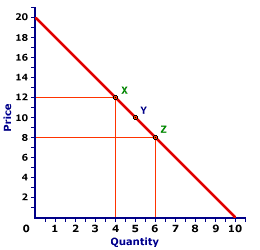Tuesday  May 30, 2023
 AmosWEB means Economics with a Touch of Whimsy!TR: The abbreviation for total revenue, which is the revenue received by a firm for the sale of its output. Total revenue is one of two parts a firm needs for the calculation of economic profit, the other is total cost. In general, total revenue is the price received for selling a good times the quantity of the good sold at that price. For a perfectly competitive firm, which receives a single unchanging price for all output sold, the calculation is relatively easy. For other real world firms, that charge different prices to different buyers for different quantities, the calculation can be more complex.Total revenue is very important in the analysis a firm's short-run production decision. Two other revenue measures directly related to total cost are average revenue and marginal revenue. Total revenue is often depicting as the total revenue curve. For a perfectly competitive firm, the total revenue curve is a straight line from the origin. For a monopoly, oligopoly, or monopolistically competitive firm, the total revenue curve is "hump-shaped," increasing at a decreasing rate, reaching a peak, then declining.ARC ELASTICITY:

The average elasticity for discrete changes in two variables. The distinguishing characteristic of arc elasticity is that percentage changes are calculated based on the average of initial and ending values of each variable, rather than initial values. Arc elasticity is generally calculated using the midpoint elasticity formula. The contrast to arc elasticity is point elasticity. For infinitesimally small changes in two variables, arc elasticity is the same as point elasticity.
Arc elasticity is best considered the average elasticity over a range of values for a relation. Like any average, some values within the range are likely to be greater and some less. However, it provides a quick approximation of elasticity when more precise and sophisticated calculation techniques are not possible.

### Working Through an Example

A Standard Demand CurveThe demand curve displayed to the right can be used to illustrate the measurement of arc elasticity using the midpoint elasticity formula. If the price declines from \$12 to \$8, the quantity demanded increases from 4 to 6, from point X to point Z. Using this midpoint formula (with price designated as P and quantity designated as Q) average price elasticity of demand is:
 midpointelasticity = (Q[Z] - Q[X])(Q[Z] + Q[X])/2 ÷ (P[Z] - P[X])(P[Z] + P[X])/2

 midpointelasticity = (6 - 4)(6 + 4)/2 ÷ (8 - 12)(8 + 12)/2 = (2)(5) ÷ (-4)(10)

 midpointelasticity = 0.4 ÷ -0.4 = -1

Ignoring the minus sign, the price elasticity of demand over this segment of the demand curve from X to Z is 1.0.

### An Average Value

This value of 1.0 is actually an average for the entire range between points X and Z. Precise estimates of point elasticity shows that the elasticity is 0.67 at point X and 1.5 at point Z. Moreover, the elasticity is different at each point on a straight line demand curve such as this one. The only point in which the elasticity is exactly equal to 1.0 is at point Y, the midpoint between X and Z.

This last observation is worth emphasizing. The midpoint elasticity formula effectively estimates the point elasticity at the very midpoint of the overall segment. This means that the elasticity of any point on a demand curve (point elasticity) can be obtained by calculating the arc elasticity with the midpoint elasticity formula such that the desired point is dead center in the middle, the midpoint of the arc.

 <= AMERICAN ECONOMIC ASSOCIATION ASSUMPTION =>Recommended Citation:

ARC ELASTICITY, AmosWEB Encyclonomic WEB*pedia, http://www.AmosWEB.com, AmosWEB LLC, 2000-2023. [Accessed: May 30, 2023].

Check Out These Related Terms...

Or For A Little Background...

And For Further Study...
Search Again?BROWN PRAGMATOX[What's This?] Today, you are likely to spend a great deal of time flipping through mail order catalogs hoping to buy either a square lamp shade with frills along the bottom or an electric coffee pot with automatic shutoff. Be on the lookout for rusty deck screws.Your Complete ScopeOkun's Law posits that the unemployment rate increases by 1% for every 2% gap between real GDP and full-employment real GDP."Never let the fear of striking out get in your way. "-- Babe RuthYIncome, Nominal Gross National ProductA PEDestrian's Guide Xtra CreditTell us what you think about AmosWEB. Like what you see? Have suggestions for improvements? Let us know. Click the User Feedback link.| | | | | | | | | | |
| | | |

Thanks for visiting AmosWEB